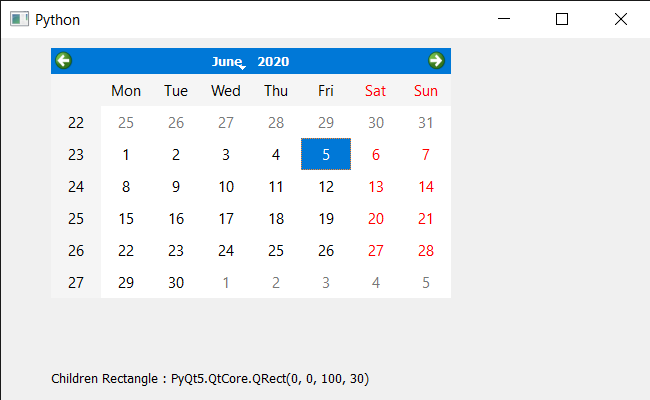# PyQt5 QCalendarWidget – Accessing Children Rectangle

• Last Updated : 06 Nov, 2021

In this article, we will see how we get the children rectangle of the QCalendarWidget. Calendar is not alone a single widget it is a mixture of lots of smaller widget which we refer as calendar’s child. There are many child such as table view, item delegate etc., we use children method to get all the children of it. Children rectangle is the bounding rectangle of all the children except hidden children.

In order to do this we will use childrenRect method with the QCalendarWidget object.
Syntax : calendar.childrenRect()
Argument : It takes no argument
Return : It return QRect object

Below is the implementation

## Python3

 `# importing libraries``from` `PyQt5.QtWidgets ``import` `*``from` `PyQt5 ``import` `QtCore, QtGui``from` `PyQt5.QtGui ``import` `*``from` `PyQt5.QtCore ``import` `*``import` `sys`  `class` `Window(QMainWindow):` `    ``def` `__init__(``self``):``        ``super``().__init__()` `        ``# setting title``        ``self``.setWindowTitle(``"Python "``)` `        ``# setting geometry``        ``self``.setGeometry(``100``, ``100``, ``650``, ``400``)` `        ``# calling method``        ``self``.UiComponents()` `        ``# showing all the widgets``        ``self``.show()` `    ``# method for components``    ``def` `UiComponents(``self``):` `        ``# creating a QCalendarWidget object``        ``self``.calendar ``=` `QCalendarWidget(``self``)` `        ``# setting geometry to the calendar``        ``self``.calendar.setGeometry(``50``, ``10``, ``400``, ``250``)` `        ``# creating a label``        ``label ``=` `QLabel(``self``)` `        ``# setting geometry``        ``label.setGeometry(``50``, ``280``, ``420``, ``120``)` `        ``# making it multi line``        ``label.setWordWrap(``True``)` `        ``# getting children rectangle``        ``value ``=` `self``.calendar.childrenRect()` `        ``# setting text to the label``        ``label.setText(``"Children Rectangle : "` `+` `str``(value))`   `# create pyqt5 app``App ``=` `QApplication(sys.argv)` `# create the instance of our Window``window ``=` `Window()`  `# start the app``sys.exit(App.``exec``())`

Output :My Personal Notes arrow_drop_up